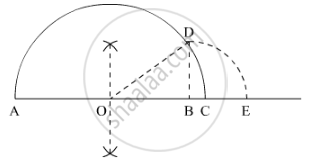# Represent Sqrt3.5, Sqrt9.4, Sqrt10.5 on the Real Number Line. - Mathematics

Represent sqrt3.5, sqrt9.4, sqrt10.5 on the real number line.

#### Solution

We are asked to represent the real numbers sqrt3.5, sqrt9.4 and sqrt10.5on the real number line

We will follow a certain algorithm to represent these numbers on real number line(a) sqrt3.5

We will take A as reference point to measure the distance

(1) Draw a sufficiently large line and mark a point A on it

(2) Take a point B on the line such that AB = 3.5 cm

(3) Mark a point C on the line such that BC = 1 cm

(4) Find mid point of AB and let it be O

(5) Take O as center and OC as radius and draw a semi circle. Draw a perpendicular BD which cuts the semi circle at D

(6) Take B as the center and BD as radius, draw an arc which cuts the horizontal line at E

(7) Point E is the representation of sqrt3.5

(b) sqrt9.4

We will take A as reference point to measure the distance. We will follow the same figure in the part (a)

(1) Draw a sufficiently large line and mark a point A on it

(2) Take a point B on the line such that AB = 9.4 cm

(3) Mark a point C on the line such that BC = 1 cm

(4) Find mid point of AB and let it be O

(5) Take O as center and OC as radius and draw a semi circle. Draw a perpendicular BC which cuts the semi circle at D

(6) Take B as the center and BD as radius, draw an arc which cuts the horizontal line at E

(7) Point E is the representation of sqrt9.4

(c) sqrt10.5

We will take A as reference point to measure the distance. We will follow the same figure in the part (a)

(1) Draw a sufficiently large line and mark a point A on

(2) Take a point B on the line such that AB = 10.5 cm

(3) Mark a point C on the line such that BC = 1 cm

(4) Find mid point of AB and let it be O

(5) Take O as center and OC as radius and draw a semi circle. Draw a perpendicular BC which cuts the semi circle at D

(6) Take B as the center and BD as radius, draw an arc which cuts the horizontal line at E

(7) Point E is the representation of sqrt10.5

Concept: Representing Real Numbers on the Number Line
Is there an error in this question or solution?
Chapter 1: Number Systems - Exercise 1.5 [Page 36]

#### APPEARS IN

RD Sharma Mathematics for Class 9
Chapter 1 Number Systems
Exercise 1.5 | Q 4 | Page 36

Share